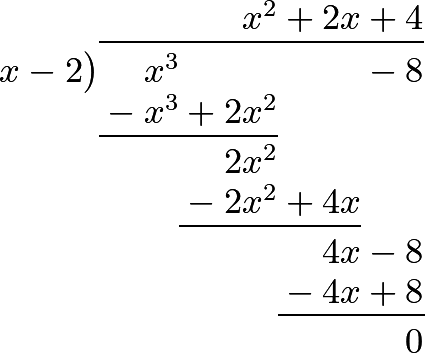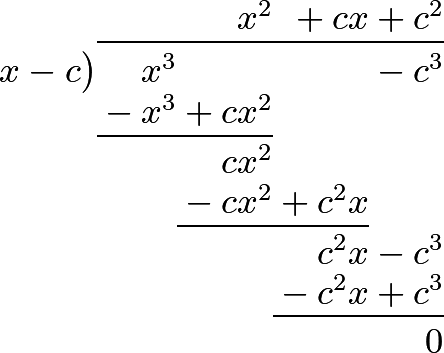### Part I. Section IV. Chapter 10. “Of Pure Equations of the Third Degree.”

706 An equation of the third degree is said to be pure, when the cube of the unknown quantity is equal to a known quantity, and when neither the square of the unknown quantity, nor the unknown quantity itself, is found in the equation; so that $$x^3=125$$, or, more generally, $$x^3=a$$, $$x^3=\dfrac{a}{b}$$, etc. are equations of this kind.

707 It is evident how we are to deduce the value of $$x$$ from such an equation, since we have only to extract the cube root of both sides. Thus, the equation $$x^3=125$$ gives $$x=5$$, the equations $$x^3=a$$ gives $$x=\sqrt{\vphantom{a}}a$$, and the equation $$x^3=\dfrac{a}{b}$$ gives $$x=\sqrt{\vphantom{\dfrac{a}{b}}}\dfrac{a}{b}$$, or $$x=\dfrac{\sqrt{\vphantom{a}}a}{\sqrt{\vphantom{b}}b}$$. To be able, therefore, to resolve such equations, it is sufficient that we know how to extract the cube root of a given number.

708 But in this manner, we obtain only one value for $$x$$: but since every equation of the second degree has two values, there is reason to suppose that an equation of the third degree has also more than one value. It will be deserving our attention to investigate this; and, if we find that in such equations $$x$$ must have several values, it will be necessary to determine those values.

709 Let us consider, for example, the equation $$x^3=8$$, with a view of deducing from it all the numbers, whose cubes are, respectively, 8. As $$x=2$$ is undoubtedly such a number, what has been said in the last chapter shows that the quantity $$x^3-8=0$$, must be divisible by $$x-2$$: let us therefore perform this division.Hence it follows, that our equation, $$x^3=8$$, may be represented by these factors;

$(x-2)(x^2+2x+4)=0.$

710 Now, the question is, to know what number we arc to substitute instead of $$x$$, in order that $$x^3=8$$, or that $$x^3-8=0$$; and it is evident that this condition is answered, by supposing the product which we have just now found equal to 0; but this happens, not only when the first factor $$x-2=0$$, which gives us $$x=2$$, but also when the second factor $$x^2+2x+4=0$$. Let us, therefore, make $$x^2+2x+4=0$$; then we shall have $$x^2=-2x-4$$, and thence $$x=-1 \pm \surd -3$$.

711 So that beside the case, in which $$x=2$$, which corresponds to the equation $$x^3=8$$, we have two other values of $$x$$, the cubes of which are also 8; and these are,

$\textrm{I)} \quad x= -1+\surd -3,\qquad \textrm{II)} \quad x= -1-\surd -3,$

as will be evident, by actually cubing these expressions

$\begin{array}{rrr} -1&+\surd -3&\\ -1&+\surd -3&\\ \hline 1&-\surd -3&\\ &-\surd -3&-3\\ \hline -2&-2\surd -3 \end{array}$
$\begin{array}{rrr} -2&-2\surd -3&\\ -1&+\surd -3&\\ \hline 2&+2\surd -3&\\ &-2\surd -3&+6\\ \hline 8&& \end{array}$
$\begin{array}{rrr} -1&-\surd -3&\\ -1&-\surd -3&\\ \hline 1&+\surd -3&\\ &+\surd -3&-3\\ \hline -2&+2\surd -3& \end{array}$
$\begin{array}{rrr} -2&+2\surd -3&\\ -1&-\surd -3&\\ \hline 2&-2\surd -3&\\ &+2\surd -3&+6\\ \hline 8&& \end{array}$

It is true, that these values are imaginary, or impossible; but yet they deserve attention.

712 What we have said applies in general to every cubic equation, such as $$x^3=a$$; namely, that beside the value $$x=\sqrt{\vphantom{a}}a$$, we shall always find two other values. To abridge the calculation, let us suppose $$\sqrt{\vphantom{a}}a=c$$, so that $$a=c^3$$, our equation will then assume this form, $$x^3-c^3=0$$, hich will be divisible by $$x-c$$, as the actual division showsConsequently, the equation in question may be represented by the product $$(x-c)(x^2+cx+c^2)=0$$, which is in fact equal to 0, not only when $$x-c=0$$, or $$x=c$$, but also when $$x^2+cx+c^2=0$$. Now, this expression contains two other values of $$x$$; for it gives $$x^2=-cx-c^2$$, and $$x=-\frac{c}{2} \pm \surd \left(\frac{c^2}{4}-c^2\right)$$, or $$x=\dfrac{-c \pm \surd -3c^2}{2}$$; that is to say,

$x=\dfrac{-c \pm c \surd -3}{2} = \dfrac{-1 \pm \surd -3}{2} \cdot c$

713 Now, as $$c$$ was substituted for $$\sqrt{\vphantom{a}}a$$, we conclude, that every equation of the third degree, of the form $$x^3=a$$, furnishes three values of $$x$$ expressed in the following manner:

1. $$x=\sqrt{\vphantom{a}}a$$,
2. $$x=\dfrac{-1 + \surd -3}{2} \cdot \sqrt{\vphantom{a}}a$$,
3. $$x=\dfrac{-1 - \surd -3}{2} \cdot \sqrt{\vphantom{a}}a$$.

This shows, that every cube root has three different values; but that one only is real, or possible, the two others being impossible. This is the more remarkable, since every square root has two values, and since we shall afterwards see, that a biquadratic root has four different values, that a fifth root has five values, and so on.

In ordinary calculations, indeed, we employ only the first of those values, because the other two are imaginary; as we shall show by some examples.

714 Question 1. To find a number, whose square, multiplied by its fourth part, may produce 432.

Let $$x$$ be that number; the product of $$x^2$$ by $$\frac{1}{4}x$$ must be equal to the number 432, that is to say, $$\frac{1}{4}x^3=432$$, and $$x^3=1728$$: whence, by extracting the cube root, we have $$x = 12$$.

The number sought therefore is 12; for its square 144, multiplied by its fourth part, or by 3, gives 432.

715 Question 2. Required a number such, that if we divide its fourth power by its half, and add 14¼ to the product, the sum may be 100.

Calling that number $$x$$, its fourth power will be $$x^4$$; dividing by the half, or $$\frac{1}{2}x$$, we have $$2x^3$$; and adding to that 14¼, the sum must be 100. We have therefore $$2x^3+14\frac{1}{4}=100$$; subtracting 14¼, there remains $$2x^3=\frac{343}{4}$$; dividing by 2, gives $$x^3=\frac{343}{8}$$, and extracting the cube root, we find $$x=\frac{7}{2}$$.

716 Question 3. Some officers being quartered in a country, each commands three times as many horsemen, and twenty times as many foot-soldiers, as there are officers. Also a horseman’s monthly pay amounts to as many florins as there are officers, and each foot-soldier receives half that pay; the whole monthly expense is 13000 florins. Required the number of officers.

If $$x$$ be the number required, each officer will have under him $$3x$$ horsemen and $$20x$$ foot-soldiers. So that the whole number of horsemen is $$3x^2$$, and that of foot-soldiers is $$20x^2$$.

Now, each horseman receiving $$x$$ florins per month, and each foot-soldier receiving $$\frac{1}{2}x$$, florins, the pay of the horsemen, each month, amounts to $$3x^3$$, and that of the footsoldiers to $$10x^3$$; consequently, they all together receive $$13x^3$$ florins, and this sum must be equal to 13000 florins: we have therefore $$13x^3=13000$$, or $$x^3=1000$$, and $$x=10$$, the number of officers required.

717 Question 4. Several merchants enter into partnership, and each contributes a hundred times as many sequins as there are partners; they send a factor to Venice, to manage their capital, who gains, for every hundred sequins, twice as many sequins as there are partners, and he returns with 2662 sequins profit. Required the number of partners.

If this number be supposed $$=x$$, each of the partners will have furnished $$100x$$ sequins, and the whole capital must have been $$100x^2$$; now, the profit being $$2x$$ for 100, the capital must have produced $$2x^3$$; so that $$2x^3=2662$$, or $$x^3 = 1331$$; this gives $$x=11$$, which is the number of partners.

718 Question 5. A country girl exchanges cheeses for hens, at the rate of two cheeses for three hens; which hens lay each ½ as many eggs as there are cheeses. Farther, the girl sells at market nine eggs for as many sous as each hen had laid eggs, receiving in all 72 sous: how many cheeses did she exchange?

Let the number of cheeses $$= x$$, then the number of hens, which the girl received in exchange, will be $$\frac{3}{2}x$$, and each hen laying $$\frac{1}{2}x$$ eggs, the number of eggs will be $$=\frac{3}{4}x^2$$. Now, as nine eggs sell for $$\frac{1}{2}x$$ sous, the money which $$\frac{3}{4}x^2$$ eggs produce is $$\frac{1}{24}x^3$$, and $$\frac{1}{24}x^3=72$$. Consequently,

$x^3=24\cdot 72=8 \cdot 3 \cdot 8 \cdot 9 = 8 \cdot 8 \cdot 27,$

whence $$x=12$$; that is to say, the girl exchanged twelve cheeses for eighteen hens.

#### Editions

1. Leonhard Euler. Elements of Algebra. Translated by Rev. John Hewlett. Third Edition. Longmans, Hurst, Rees, Orme, and Co. London. 1822.
2. Leonhard Euler. Vollständige Anleitung zur Algebra. Mit den Zusätzen von Joseph Louis Lagrange. Herausgegeben von Heinrich Weber. B. G. Teubner. Leipzig and Berlin. 1911. Leonhardi Euleri Opera omnia. Series prima. Opera mathematica. Volumen primum.